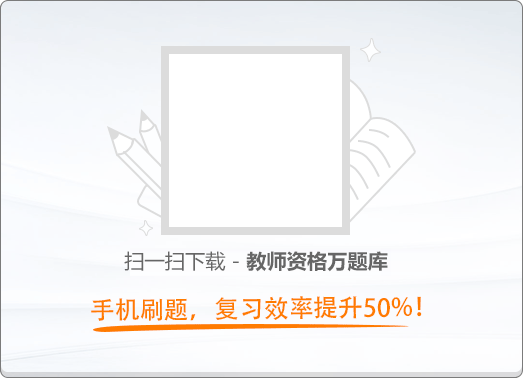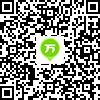120:002019年11月《幼儿综合素质》真题

1
(单项选择题)

• A.

教师要引导幼儿正确认知

• B.

教师要关注幼儿的特长

• C.

教师要引导幼儿，进行生涯规划

• D.

教师要关注幼儿的差异

• A
• B
• C
• D

2
(单项选择题)

• A.

注重幼儿知识积累

• B.

注重幼儿气质养成

• C.

注重幼儿情境体验

• D.

注重幼儿习惯培养

• A
• B
• C
• D

3
(单项选择题)

• A.

怎么这么不小心

• B.

没关系吧，需要帮助吗

• C.

来，我扶你起来

• D.

赶紧起来，勇敢点儿

• A
• B
• C
• D

4
(单项选择题)

• A.

通知家长领回训练

• B.

统一要求，不能特殊

• C.

批评点点坚持常规地

• D.

降低要求个别对待

• A
• B
• C
• D

5
(单项选择题)• A.

体现了对孩子的严格要求

• B.

有助于激励孩子不断进步

• C.

损害了孩子的人格尊严

• D.

侵犯了孩子的受教育权

• A
• B
• C
• D

6
(单项选择题)

《中华人民共和国宪法》规定，任何组织或者个人的权利都不得超越（　）。

• A.

宪法和法规

• B.

宪法和法律

• C.

法律和法规

• D.

政策和法律

• A
• B
• C
• D

7
(单项选择题)

• A.

幼儿园的规模一般不超过500人

• B.

入园幼儿只能由法定监护人接送

• C.

幼儿一日活动的组织应当动静交替

• D.

幼儿入园可进行健康检查和认知测查

• A
• B
• C
• D

8
(单项选择题)

• A.

应由公安机关给予治安管理处罚

• B.

应由教育行政部门给予行政处罚

• C.

应由人民法院给予司法拘留

• D.

应由人民检察院给予刑事处罚

• A
• B
• C
• D

9
(单项选择题)

• A.

民事处罚

• B.

行政处分

• C.

刑事处罚

• D.

行政处罚

• A
• B
• C
• D

10
(单项选择题)

• A.

正确。有助于督促幼儿习惯养成

• B.

正确。有助于激发幼儿积极表现

• C.

不正确。侵犯了幼儿家长的荣誉权

• D.

不正确。侵犯了幼儿家长的财产权

• A
• B
• C
• D

11
(单项选择题)

• A.

林某应继续负担抚养费用

• B.

林某可不再承担抚养费用

• C.

法院可委托他人代为监护

• D.

林某可指定他人代为监护

• A
• B
• C
• D

12
(单项选择题)

• A.

合法，幼儿园有权利处理幼儿课堂画作

• B.

合法，任何人不得干涉幼儿园的决定

• C.

不合法，幼儿园侵犯了兰兰的财产权

• D.

不合法，幼儿园侵犯了兰兰的著作权

• A
• B
• C
• D

13
(单项选择题)

• A.

具有心理调适能力

• B.

具有反思意识

• C.

具有心理干预能力

• D.

具有艺术造诣

• A
• B
• C
• D

14
(单项选择题)

• A.

教师劳动具有专业性

• B.

教师劳动具有创造性

• C.

教师劳动具有复杂性

• D.

教师劳动具有重复性

• A
• B
• C
• D

15
(单项选择题)

• A.

幼儿发展是能动的

• B.

幼儿发展是平衡的

• C.

幼儿发展是平等的

• D.

幼儿发展是持续的

• A
• B
• C
• D

16
(单项选择题)

• A.

“祸患常积于忽微，而智勇多困于所溺。”

• B.

“不要人夸颜色好，只留清气满乾坤。”

• C.

“明者因时而变，知者随事而制。”

• D.

“善禁者，先禁其身，而后人。”

• A
• B
• C
• D

17
(单项选择题)

• A.

雷锋

• B.

孟泰

• C.

焦裕禄

• D.

王进喜

• A
• B
• C
• D

18
(单项选择题)

• A.

• B.

• C.

• D.

• A
• B
• C
• D

19
(单项选择题)

• A.

《黄帝内经》

• B.

《伤寒杂病论》

• C.

《千金方》

• D.

《本草纲目》

• A
• B
• C
• D

20
(单项选择题)

• A.

柴达木盆地

• B.

四川盆地

• C.

吐鲁番盆地

• D.

塔里木盆地

• A
• B
• C
• D

21
(单项选择题)

1979年，我国第一艘航天测量船投入使用，成为世界上第四个拥有远洋航天测量船的国家，该航天测量船名称是（　）。

• A.

大洋一号

• B.

远望一号

• C.

东力红一号

• D.

向阳红一号

• A
• B
• C
• D

22
(单项选择题)

“冬不穿白，夏不穿黑”，是人们在实践中总结出来的生活经验。它体现的科学常识是者（　）。

• A.

太阳光的吸收与反射

• B.

冬夏雨水的变化规律

• C.

颜色搭配的视觉效果

• D.

冬夏景物的巨大变化

• A
• B
• C
• D

23
(单项选择题)

• A.

自来水管内水的流动声

• B.

自来水管与流动的水碰撞

• C.

水龙头拧紧阻碍了水流动

• D.

水冲出时与水龙头共振

• A
• B
• C
• D

24
(单项选择题)

《搜神记》是魏晋南北朝志怪小说中最完整、最有代表性的作品集。它汇集了晋朝前民间传说中的神奇怪异故事，很多故事都具有比较积极的意义，对后世影响深远。下列选项中，不是出自《搜神记》的故事是（　）。

• A.

牛郎织女

• B.

李寄斩蛇

• C.

干将莫邪

• D.

吴王小女

• A
• B
• C
• D

25
(单项选择题)• A.

A

• B.

B

• C.

C

• D.

D

• A
• B
• C
• D

26
(单项选择题)

• A.

首行缩进

• B.

悬挂缩进

• C.

左缩进

• D.

右缩进

• A
• B
• C
• D

27
(单项选择题)

• A.

排练计时

• B.

自边放映

• C.

批注功能

• D.

使用母版

• A
• B
• C
• D

28
(单项选择题)

• A.

土豆与马铃薯

• B.

桃子与水蜜桃

• C.

芒果与火龙果

• D.

萝卜与红萝卜

• A
• B
• C
• D

29
(单项选择题)• A.

A

• B.

B

• C.

C

• D.

D

• A
• B
• C
• D